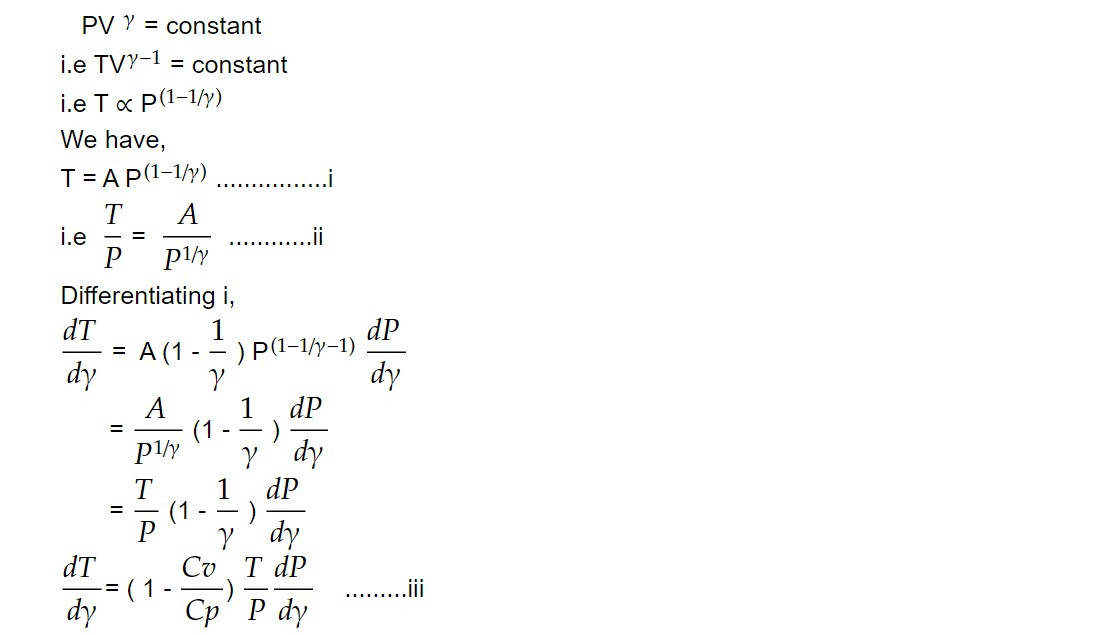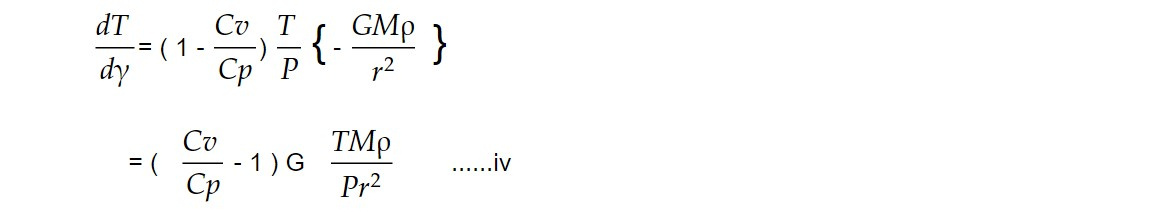# Energy transfer mechanism

Local thermodynamical equilibrium:

Thermodynamical equilibrium between the gases (neutral, partially ionized, completely ionized) and radiation in which Maxwellian velocity distribution (neutral gas), Boltzmann distribution (partially ionized gas), Saha distribution (completely ionized gas ) and Plank theory of black radiation holds is called local thermodynamical equilibrium.

Let us review these distribution

1. Maxwellian velocity distribution:

If the neutral gas (particles) is in thermodynamic equilibrium at a particular temperature T, then the number of particles having speeds between v and v+dv is given byHere n is the number density and m is the mass of each particle.

2. Boltzmann distribution:

If no is the number density of atoms in the ground state, then the number density ne of atoms in an excited state with energy E above the ground state is given by

ne/no = exp {-E/ KT} .......2

where E is excitation energy which is discrete in nature.

3. Saha distribution:

Saha (1920) derived an equation which tells us what fraction of gas will be ionised at a particular temperature T and pressure P. If Φ is the ionization potential, then the fraction x of atom which are ionized is given by4. Planck's law:

When the radiation is in thermodynamic equilibrium with the matter is called black body radiation. The energy density of electromagnetic radiation when it is in the thermodynamic equilibrium is given bySince black body radiation is isotropic we do not need to provide directional information. The specific intensity or Planck function of black body radiation isIf the matter is present, then in general specific intensity keeps changing as we move along a ray path.

LTE is the situation under which gases and radiation obey all four laws (eq i -iv) at a particular temperature.

The condition for LTE are as follows

i. The temperature should not vary within the mean free path of gases (neutral, ionized, partially ionized)

ii. The reciprocal of absorption coefficient (µ) of photon should be of the order of mean free path of gas.

Conduction:

The energy transport via conduction is possible only when the gases carry energy and transport it via atomic or molecular excitation. In the star, gases are mostly ionized. The only possibility is that the electron (free) itself carry energy and transport it. But the electron carrying energy can travel only a short distance without colliding other particle. Thus, conductive energy transport in the normal star is negligible (< 0.001 %).

Convection:

If the star has a high opacity (high density) in a particular region, photon can travel only a limit distance before being absorbed or scattered. In this situation random walk will be excessively slow. In other words, photons get trapped in a particular region due to which temperature continuously increases. In this situation, the hot region behaves as a riding bubble and pushes the stellar material outwards.

When the temperature gradient becomes very large, the medium begins to convert. The hot region (rising bubble) pushes the gases to move outwards obeying adiabatic equation of state. For reversible adiabatic process. We knowThus convective temperature gradient is proportional to convective pressure gradient.

Using hydrostatic equilibrium we can write,We know that ρ, M, P and T run with r. Thus, the density profile should be known to solve this equation. Here the density profile means the change in the density in this convective gradient isWe know that for a highly ionized plasma state, ratio of specific heat capacity γ = 5/3. The value of γ depends on the degree of ionization of gases and chemical composition.

This note is taken from Astrophysics, MSC Nepal.

This note is a part of the Physics Repository.